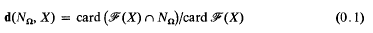Skip to main content Accessibility help
Home

# The Farey density of norm subgroups in global fields (I)

## Extract

Let Ω be an algebraic number field, and let NΩ ⊂ ℚ be the group of norms of fractional ideals of Ω. Then NΩ is a subgroup of the positive rationals; the latter is the direct sum of a denumerable infinity of infinite cyclic groups, and so it is free abelian; thus NΩ is free abelian, and, since it is not finitely generated, we must havequa abstract groups. The purpose of this paper is, in the first place, to find a “metrical ” way of distinguishing these isomorphic groups, and, to this end, we introduce the notion of Farey density, defined as follows; let X be a positive integer, and consider the Farey section ℱ(X) of order X, thus the set of all reduced positive fractions with denominator < X; then the quotientmeasures the proportion of elements of ℱ(X) which are in NΩ, and, as X → ∞, it gives a measure of the “density ” of fractional ideal norms in the rational interval (0, 1).

## References

Hide All
MathJax
MathJax is a JavaScript display engine for mathematics. For more information see http://www.mathjax.org.

## Metrics

### Full text viewsFull text views reflects the number of PDF downloads, PDFs sent to Google Drive, Dropbox and Kindle and HTML full text views.

Total number of HTML views: 0
Total number of PDF views: 0 *Loading metrics...

### Abstract viewsAbstract views reflect the number of visits to the article landing page.

Total abstract views: 0 *Loading metrics...

* Views captured on Cambridge Core between <date>. This data will be updated every 24 hours.

Usage data cannot currently be displayed